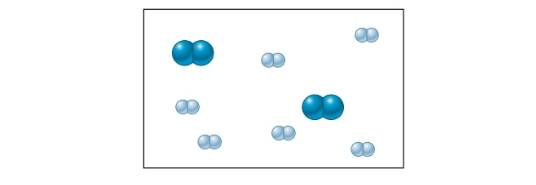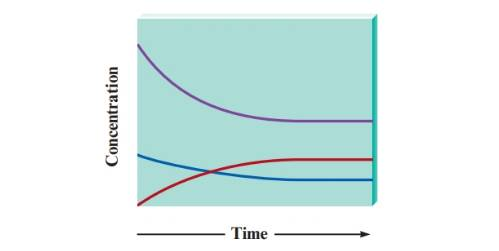Chapter 17, Problem 12QAP### Introductory Chemistry: A Foundati...

9th Edition
Steven S. Zumdahl + 1 other
ISBN: 9781337399425

#### Solutions

Chapter
Section### Introductory Chemistry: A Foundati...

9th Edition
Steven S. Zumdahl + 1 other
ISBN: 9781337399425
Textbook Problem
121 views

# . Consider an initial mixture of N2 and H2 gases that can be represented as follows:The gases react to form ammonia gas (NH3) as represented by the following concentration profile:a. Label each plot on the graph as N2, H2, or NH3, and explain your answers. b. Explain the relative shapes of the plots. c. When is equilibrium reached? How do you know?

Interpretation Introduction

(a)

Interpretation:

Each plot on the graph is to be labelled as N2, H2 or NH3 and an explanation for the same is to be stated.

Concept Introduction:

The chemical equilibrium is the state in which the rates of both forward and backward reactions become equal. The concentration of both reactants and products do not change on reaching an equilibrium state.

The general chemical equation is shown below.

aA+bBcC+dD.

Explanation

In the graph, red curve is started from zero due to which, it must be the product of a reaction. It is given that the gases react to produce ammonia gas. Hence, the red curve represents ammonia gas in a reaction.

An initial mixture of N2 and H2 gases has been taken for the formation of ammonia gas. The balanced chemical equation of N2 and H2 to form NH3 is shown below

Interpretation Introduction

(b)

Interpretation:

The explanation for the relative shapes of the plots is to be stated.

Concept Introduction:

The chemical equilibrium is the state in which the rates of both forward and backward reactions become equal. The concentration of both reactants and products do not change on reaching an equilibrium state.

The general chemical equation is shown below.

aA+bBcC+dD.

Interpretation Introduction

(c)

Interpretation:

The stage at which equilibrium is reached in a given reaction and the explanation for the same are to be stated.

Concept Introduction:

The chemical equilibrium is the state in which the rates of both forward and backward reactions become equal. The concentration of both reactants and products do not change on reaching an equilibrium state.

The general chemical equation is shown below.

aA+bBcC+dD.

### Still sussing out bartleby?

Check out a sample textbook solution.

See a sample solution

#### The Solution to Your Study Problems

Bartleby provides explanations to thousands of textbook problems written by our experts, many with advanced degrees!

Get Started